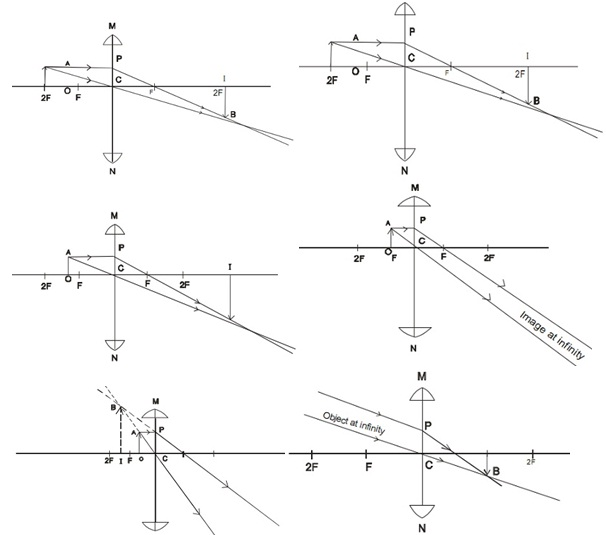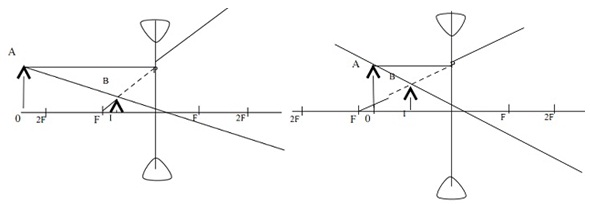#### Refraction at Curved Surfaces, Physics tutorial

Refraction through Lenses:

Refraction via lenses comprises the change in direction of light rays. A lens is any transparent material having two faces of which at least one is curved. It causes a beam of light passing via it to either converge or diverge.

Types of Lenses:

We encompass two kinds of lenses namely convex lens and concave lens.

1) A convex lens is a converging lens. It forms a parallel beam of light falling on it to converge. This is thicker at its centre than at its edges.

2) A concave lens is the diverging lens. It forms parallel beams of light falling on it to appear to diverge from a point. This is thinner at its centre than at the edges.

Rules for forming Images made by:

1) Rules for convex (converging) lens:

You will follow the given rules whenever constructing images made by convex (converging) lens.

Rule 1: Incident rays parallel to the principal axis are refracted via the principal focus.

Rule 2: Incident rays passing via the optical center are un-deviated.

Rule 3: Incident rays passing via the principal focus come out parallel to the principal axis after being refracted via the lens.

2) Rule for concave (diverging) lens:

The given rules will guide you whenever constructing images made by concave (diverging) lens.

Rule 1: The incident rays parallel to the principal axis are refracted away from the principal focus.

Rule 2: The incident rays passing via the optical centre are un-deviated.

Formation of Images by Lenses:

We must note that light rays incident on the converging lens converge to make a real image on the other side of the lens after passing via the lens. For a diverging lens, the rays from an object diverge to make a virtual image of the object. Whenever we know the distance of an object from the lens and the focal length of the lens, we can get the position and nature of the image by means of the construction of ray's diagrams.

1) Formation of Images by a Convex (Converging) Lens:a) Object between F and Lens image is: Erect, Magnified, Virtual and beyond the object on same side as object.

b) Object at F: The image is at infinity, Real, Inverted and Magnified.

c) Object between F and 2F image is: Inverted, Magnified, Real and beyond 2f.

d) Object at 2F Image is: Inverted, Real, Same size as object and at 2F of the other side of the lens.

e) Object beyond 2f Image is: Inverted, Diminished, Real, Between F and 2F on the other side of lens.

f) Object at infinity image is: Inverted, Diminished, Real and At F on the other side of lens.

2) Formation of Images by a Concave (Diverging) Lens:(a) Object beyond 2f image is: Erect and Diminished, Virtual, Between F and C

(b) Object between F and C image is: Erect, Diminished, Virtual 2F, Between F and C

Practical Applications of the Convex (Converging) Lens:

We employ the convex (or converging) lens in numerous optical instruments. A few of them are listed as follows.

1) If the object is between F and lens, the lens is employed as eye lens of numerous instruments, magnifying glass, spectacles for long sight.

2) If object is at F, it is employed at theatre and stadium spotlights.

3) If object is between F and 2F, it is employed as objective lens of the microscopes and projectors.

4) If object is at 2F, it is utilized in copying camera.

Practical Application of the Concave (Diverging) Lens:

A concave lens is generally employed in spectacles for short sight.

Tutorsglobe: A way to secure high grade in your curriculum (Online Tutoring)

Expand your confidence, grow study skills and improve your grades.

Since 2009, Tutorsglobe has proactively helped millions of students to get better grades in school, college or university and score well in competitive tests with live, one-on-one online tutoring.

Using an advanced developed tutoring system providing little or no wait time, the students are connected on-demand with a tutor at www.tutorsglobe.com. Students work one-on-one, in real-time with a tutor, communicating and studying using a virtual whiteboard technology.  Scientific and mathematical notation, symbols, geometric figures, graphing and freehand drawing can be rendered quickly and easily in the advanced whiteboard.

Free to know our price and packages for online physics tutoring. Chat with us or submit request at info@tutorsglobe.com